# Even and Odd Numbers | Definition, Facts, and Examples

1. Math Lessons >
2. Even and Odd Numbers

This lesson is all about two broad categories of numbers in math: even and odd.

### What Are Even and Odd Numbers?

A number is even if it can be divided equally into two, and it’s odd if it can’t be split equally.

To understand the classification better, let’s take this scenario:

Greg and Jake buy 6 cupcakes.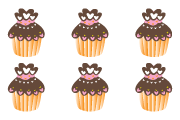Sharing the cupcakes equally, each gets 3.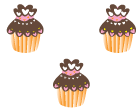Greg's shareJake's share

Since Greg and Jake could share the cupcakes equally, we know that 6 is an even number.

Now let's try sharing these 9 candies between Amanda and Sandra.After Amanda and Sandra get 4 candies each, there will be 1 candy left.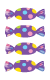Amanda's shareSandra's share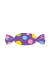Leftover

We couldn’t divide the candies equally between the two, so 9 is an odd number.

### Can You Identify Even and Odd Numbers by Pairing?

Pairing is yet another way to find out if a number is odd or even. A number is even if it can be grouped into pairs or twos, and it’s odd if it can’t be grouped into pairs.

Take these pairs of socks for instance: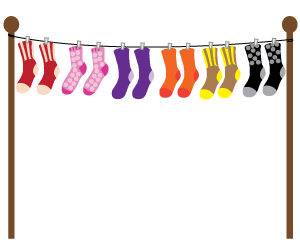We have 6 pairs of socks here, which means 12 is an even number.

Now let’s check if there are odd or even number of mittens here.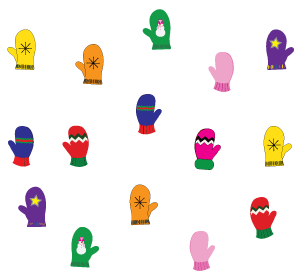Let’s put them in pairs and find out if any are left unpaired.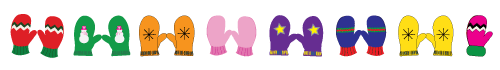We have 7 pairs and one unpaired mitten here. That shows 15 is an odd number.

### Can You Identify Even and Odd Numbers Using the Rightmost Digit?

The easiest way to identify an even or odd number is by looking at what the number ends with.

The numbers ending with 0, 2, 4, 6, or 8 are even.

The numbers ending with 1, 3, 5, 7, or 9 are odd.

If you put the numbers in order, you will sure notice a pattern.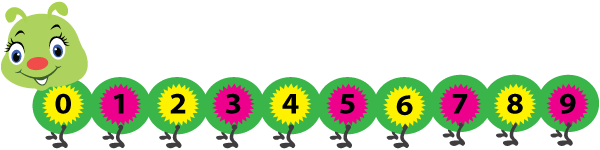Yes, odd and even numbers alternate with each other. This alternating pattern goes on and on, no matter how many digits a number has! In fact, the digits are just a combination of the numerals 0 to 9, aren’t they?

Click on the circles to spot the six even numbers

999
98
60
79
86
209
10
103
522
986
Reset

As you can see, what actually matters is the rightmost digit of a number. You don’t really have to worry about the other digits.

### Bigger Numbers This Time!

Check if the following numbers are even or odd.

1) 1,564  – Even

2) 256,789  – Odd

3) 899,251  – Odd

4) 39,857  – Odd

5) 996,966  – Even

So it’s pretty clear no matter how big the number is, this rule of thumb will definitely work.

### A Quick Recap

• If you can divide the number of items into two groups with an equal number of items in each, the number is even.

• If you end up with any leftovers on dividing the number of items into two equal groups, the number is odd.

• If you can pair up objects in a set, the set has an even number of objects. If there are any leftovers, the set has an odd number of objects.

• In a whole number sequence, even and odd numbers alternate with each other.

• A number with 0, 2, 4, 6, or 8 as the rightmost digit is even. A number with 1, 3, 5, 7, or 9 as the rightmost digit is odd.Check your prowess with our free printable Even and Odd Numbers worksheets.

Progress
Score

Restart Quiz